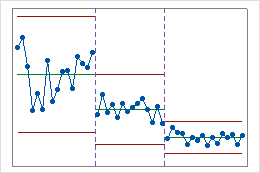# All statistics and graphs for Multivariate EWMA Chart

Find definitions and interpretation guidance for every statistic and graph that is provided with the multivariate EWMA chart.

## Plotted points

The plotted points on the Multivariate EWMA are the multivariate exponentially weighted moving averages.

### Interpretation

If the process is in control, the points fall below the upper control limit, and the process exhibits only common-cause variation. Investigate points that fall above the upper control limit. One disadvantage to multivariate charts is that the scale is unrelated to the scale of any of the variables, and out-of-control signals do not reveal which variable (or combination of variables) caused the signal.

## Control limits

The upper control limit is the horizontal line above the center line. The upper control limit indicates whether a process is out of control, and it is based on the observed variation within subgroups and on the expected variation in the plotted points.

## Covariance matrices

A covariance matrix is a square matrix that contains the variances and covariances for several variables. The diagonal elements of the matrix contain the variances of the variables, and the off-diagonal elements contain the covariances between all possible pairs of variables.

The variance-covariance matrix is symmetric because the covariance between X and Y is the same as the covariance between Y and X. Therefore, the covariance for each pair of variables appears twice in the matrix: the covariance between the ith and jth variables is displayed at positions (i, j) and (j, i).

After you store the covariance matrices, choose Data > Display Data to view the covariance matrices.

### Interpretation

 X Y Z X 2.0 −0.86 −0.15 Y −0.86 3.4 0.48 Z −0.15 0.48 0.82

The variances are displayed in bold along the diagonal. The variance of X, Y, and Z are 2.0, 3.4, and 0.82 respectively. The covariance between X and Y is −0.86, the covariance between X and Z is −0.15, and the covariance between Y and Z is 0.48.

## Stages

Use stages to create a historical control chart that shows how a process changes over specific periods of time. By default, Minitab recalculates the center line and control limits for each stage. For more information, go to Add stages to show how a process changed.

### Interpretation

This historical control chart shows three stages of a process, which represent before, during, and after the implementation of a new procedure.By using this site you agree to the use of cookies for analytics and personalized content.  Read our policy